#DAY 15
0

## 將輪廓中的顏色當作篩選指標

``````n = len(contours)
for i in range(n):
M = cv.moments(contours[i])
area = M['m00']
if area/total_area>0.15 and area/total_area<0.50:#若面積大於總面積的15%且小於50%
cx = int(M['m10']/M['m00'])
cy = int(M['m01']/M['m00'])
center = tuple((cx,cy))
if cx>img.shape*0.25 and cx<img.shape*0.75:
#將輪廓描繪在複製圖上
copy = cv.drawContours(img,contours[i],-1,(255,0,0),2)

#建立單通道mask，並計算
mask = np.zeros(gray.shape,dtype=np.uint8)
cv.drawContours(mask,contours[i],-1,(255,255,255),-1)
meanVal = cv.mean(img,mask=mask) #mask是一個區域，所以必須是單通道的
print(meanVal)#print出輪廓的B、G、R顏色平均值
``````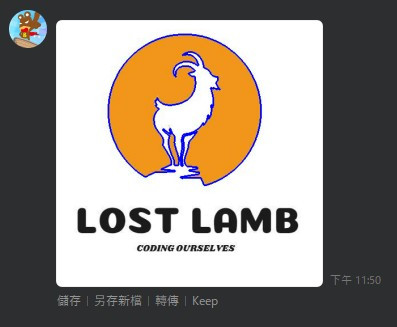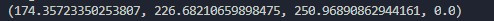``````    #將灰階圖進行二值化處理，將灰階值門檻設為220
ret,binary=cv.threshold(gray,220,255,cv.THRESH_BINARY)

#將二值化的圖片放到findContours()中
contours,hierarchy = cv.findContours(binary,cv.RETR_LIST,cv.CHAIN_APPROX_NONE)

#首先複製原圖
copy = img.copy()

#建立空白圖片
empty = np.ones(img.shape,dtype=np.uint8)*255

total_area = img.shape*img.shape
font = cv.FONT_HERSHEY_DUPLEX#設定cv字型

n = len(contours)
for i in range(n):
M = cv.moments(contours[i])
area = M['m00']
if area/total_area>0.001 and area/total_area<0.800:#若面積大於總面積的15%且小於50%
#將輪廓描繪在複製圖上
mask = np.zeros(gray.shape,dtype=np.uint8)
cv.drawContours(mask,contours[i],-1,(255,255,255),-1)
meanVal = cv.mean(img,mask=mask) #mask是一個區域，所以必須是單通道的
if meanVal>meanVal and meanVal>meanVal:
print(meanVal)#將輪廓中的平均數值print出來
if abs(meanVal-meanVal)>10:#若紅色與綠色絕對值差10以上
copy = cv.drawContours(img,contours[i],-1,(0,0,255),2)#將輪廓繪製到空白底圖中

``````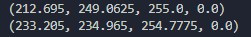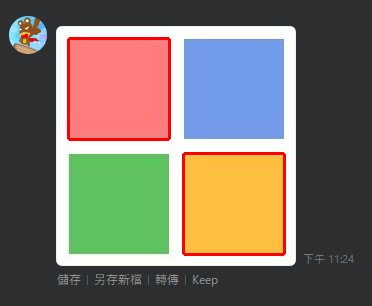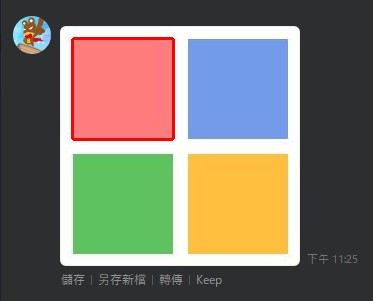ID mastermaso